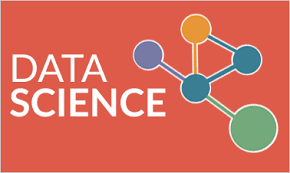21.What is Sampling and Sampling Distribution?
SAMPLING: Sampling is the process of choosing units (ex- people, organizations) from a population of interest so that by studying the sample we can fairly generalize our results back to the population from which they were chosen.
SAMPLING DISTRIBUTION: The sampling distribution of a statistic is the distribution of that statistic, considered as a random variable, when derived from a random sample of size n. It may be considered as the distribution of the statistic for all possible samples from the same population of a given size.

22.What is Linear Regression?
In statistics, linear regression is an way for modeling the relationship between a scalar dependent variable y and one or more explanatory variables (or independent variable) denoted by X. The case of one explanatory variable is known as simple linear regression.

23.Differentiate between Extrapolation and Interpolation?
Extrapolation is an approximate of a value based on extending a known sequence of values or facts beyond the area that is certainly known. Interpolation is an estimation of a value within two known values in a list of values.

24.How expected value is different from Mean value?
There is no difference. These are two names for the same thing. They are mostly used in different contexts, though if we talk about the expected value of a random variable and the mean of a sample, population or probability distribution.

25.Differentiate between Systematic and Cluster Sampling?
SYSTEMATIC SAMPLING: Systematic sampling is a statistical methology involving the selection of elements from an ordered sampling frame. The most common form of systematic sampling is an equal-probability method.
CLUSTER SAMPLING: A cluster sample is a probability sample by which each sampling unit is a collection, or cluster, of elements.

26.What are the advantages of Systematic Sampling?
1.Easier to perform in the field, especially if a proper frame is not available.
2. Regularly provides more information per unit cost than simple random sampling, in the sense of smaller variances.

27.What do you understand by term Threshold limit value?
The threshold limit value (TLV) of a chemical substance is a level in which it is believed that a worker can be exposed day after day for a working lifetime  without affecting his/her health.

28.Differentiate between Validation Set and Test set?
Validation set: It is a set of examples used to tune the parameters [i.e., architecture, not weights] of a classifier, for example to choose the number of hidden units in a neural network.
Test set: A set of examples used only to assess the performance [generalization] of a fully specified classifier.

29.How can R and Hadoop be used together?
The most common way to link R and Hadoop is to use HDFS (potentially managed by Hive or HBase) as the long-term store for all data, and use Map Reduce jobs (potentially submitted from Hive, Pig, or Oozie) to encode, enrich, and sample data sets from HDFS into R. Data analysts can then perform complex modeling exercises on a subset of prepared data in R.

30.What do you understand by term RIMPALA?
RImpala-package contains the R functions required to connect, execute queries and retrieve back results from Impala. It uses the rJava package to create a      JDBC connection to any of the impala servers running on a Hadoop Cluster.